# How to Calculate and Solve for Schottky Defect | Ceramics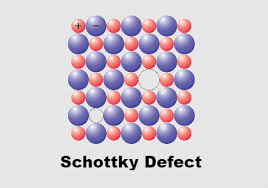The image above represents schottky defect.

To compute for schottky defect, four essential parameters are needed and these parameters are N, Activation energy (Qs), Boltzmann’s Constant (K) and Temperature (T).

The formula for calculating schottky defect:

Ns = N exp (-Qs/2KT)

Where:

Qs = Activation Energy
K = Boltzmann’s Constant
T = Temperature

Let’s solve an example;
Find the schottky defect when the activation energy is 44, N is 22, boltzmann’s constant is 1.38064852E-23 and the temperature is 30.

This implies that;

N = 22
Qs = Activation Energy = 44
K = Boltzmann’s Constant = 1.38054852E-23
T = Temperature = 30

Ns = N exp (-Qs/2KT)
Ns = (22)exp(-(44)/2(1.38064852e-23)(30))
Ns = (22)exp((-44)/(8.283891119e-22))
Ns = (22)exp(-5.3115135583771414e+22)
Ns = (22)(0)
Ns = 0

Therefore, the schottky defect is 0.

Calculating the N when the Schottky Defect, the Activation Energy, the Boltzmann’s Constant and the Temperature is Given.

N = Ns / e (-Qs / 2KT)

Where;

Ns = Schottky Defect
Qs = Activation Energy
K = Boltzmann’s Constant
T = Temperature

Let’s solve an example;
Find the N when the schottky defect is 40, the activation energy is 24, the boltzmann’s constant is 1.38064852E-23 and the temperature is 10.

This implies that;

Ns = Schottky Defect = 40
Qs = Activation Energy = 24
K = Boltzmann’s Constant = 1.38064852E-23
T = Temperature = 10

N = Ns / e (-Qs / 2KT)
N = 40 / e (-24 / 2 x 1.38064852E-23 x 10)
N = 40 / e (-24 / 2.76129704E+23)
N = 40 / e (8.691567e-23)
N = 40 / 8.691567e+23
N = 4.602e-23

Therefore, the is 4.602e-23.

Calculating the Activation Energy when the Schottky Defect, the Boltzmann’s Constant, the Temperature and N is Given.

Qs = – (In (Ns / N) x 2KT)

Where;

Qs = Activation Energy
Ns = Schottky Defect
K = Boltzmann’s Constant
T = Temperature

Let’s solve an example;
Find the activation energy when the schottky defect is 25, the boltzmann’s Constant is 1.38064852E-23, the temperature is 10 and N is 5.

This implies that;

Ns = Schottky Defect = 25
K = Boltzmann’s Constant = 1.38064852e-23
T = Temperature = 10
N = 5

Qs = – (In (Ns / N) x 2KT)
Qs = – (In (25 / 5) x 2 x 1.38064852e-23 x 10)
Qs = – (In (5) x 2.76129704e+23)
Qs = – (In 1.38064852e+24)
Qs = – 55.584

Therefore, the activation energy is – 55.584.

Calculating the Temperature when the Schottky Defect, the Activation Energy, Boltzmann’s Constant and N is Given.

T = – Qs / In (Ns / N) x 1 / 2K

Where;

T = Temperature
Qs = Activation Energy
Ns = Schottky Defect
K = Boltzmann’s Constant

Let’s solve an example;
Find the temperature when the activation energy is 44, the schottky defect is 32, the boltzmann’s constant is 1.38064852e-23 and N is 8.

This implies that;

Qs = Activation Energy = 44
Ns = Schottky Defect = 32
K = Boltzmann’s Constant = 1.38064852e-23
N = 8

T = – Qs / In (Ns / N) x 1 / 2K
T = – 44 / In (32 / 8) x 1 / 2 x 1.38064852e-23
T = – 44 / In (4) x 1 / 2.76129704e+23
T = – 44 / 1.38629 x 3.6214e-24
T = – 31.739 x 3.6214e-24
T = 1.14939e+23

Therefore, the temperature is 1.14939e+23.

Nickzom Calculator – The Calculator Encyclopedia is capable of calculating the schottky defect.

To get the answer and workings of the schottky defect using the Nickzom Calculator – The Calculator Encyclopedia. First, you need to obtain the app.

You can get this app via any of these means:

To get access to the professional version via web, you need to register and subscribe for NGN 1,500 per annum to have utter access to all functionalities.
You can also try the demo version via https://www.nickzom.org/calculator

Apple (Paid) – https://itunes.apple.com/us/app/nickzom-calculator/id1331162702?mt=8
Once, you have obtained the calculator encyclopedia app, proceed to the Calculator Map, then click on Materials & Metallurgical under Engineering.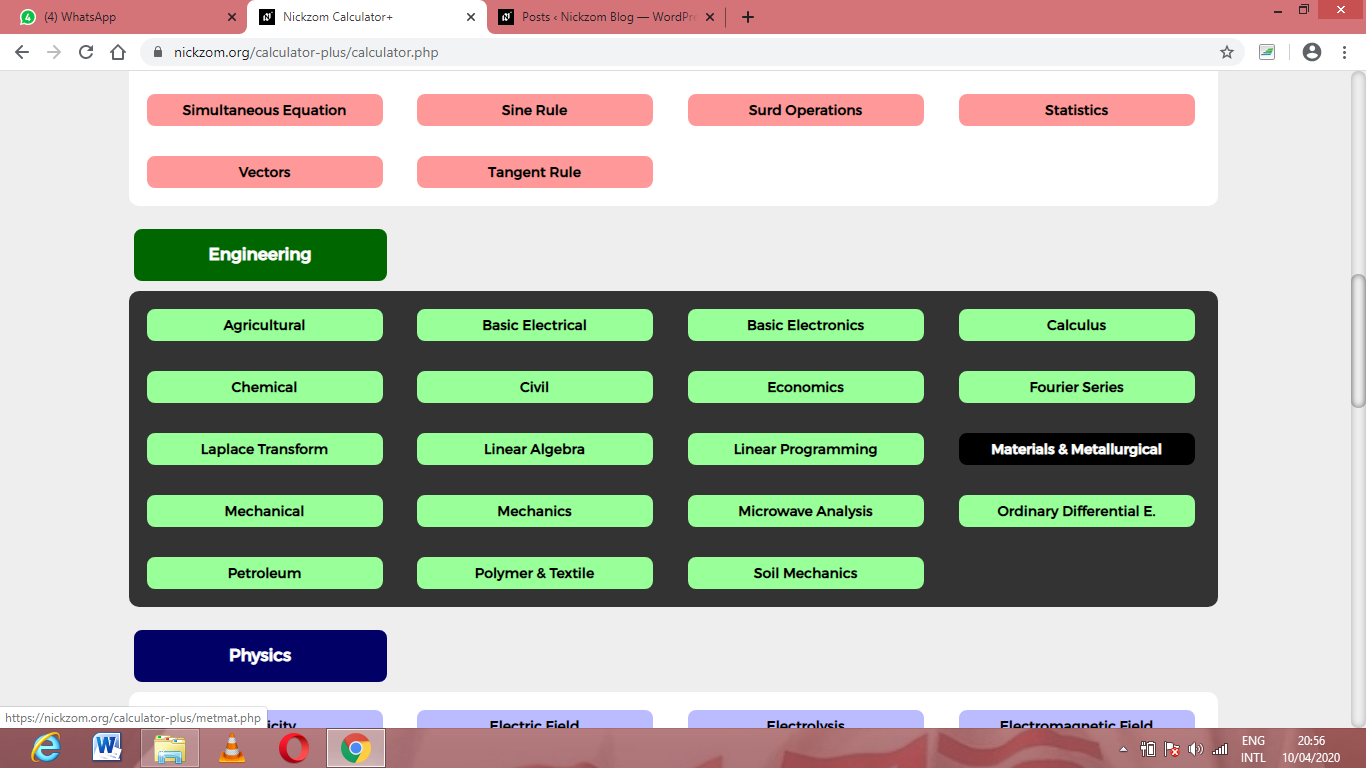Now, Click on Ceramics under Material & Metallurgical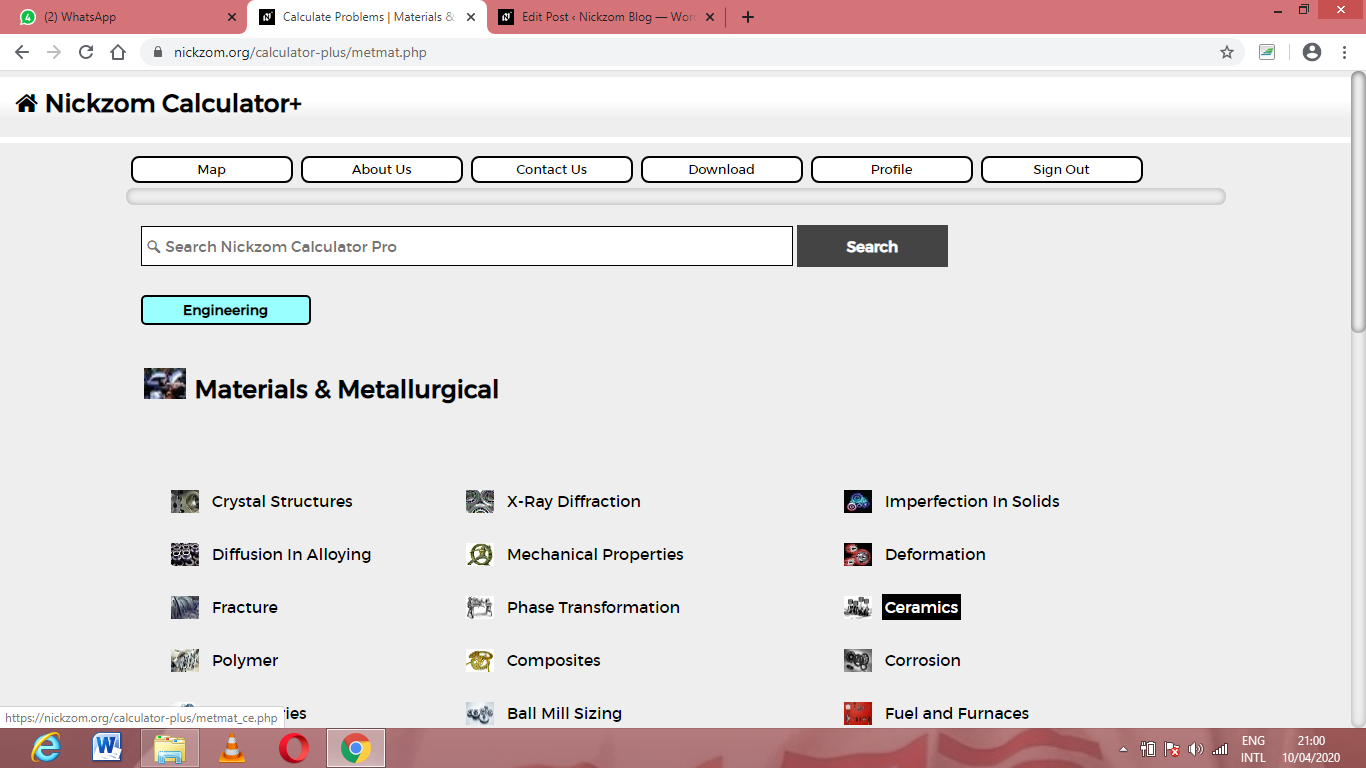Now, Click on Schottky Defect under Ceramics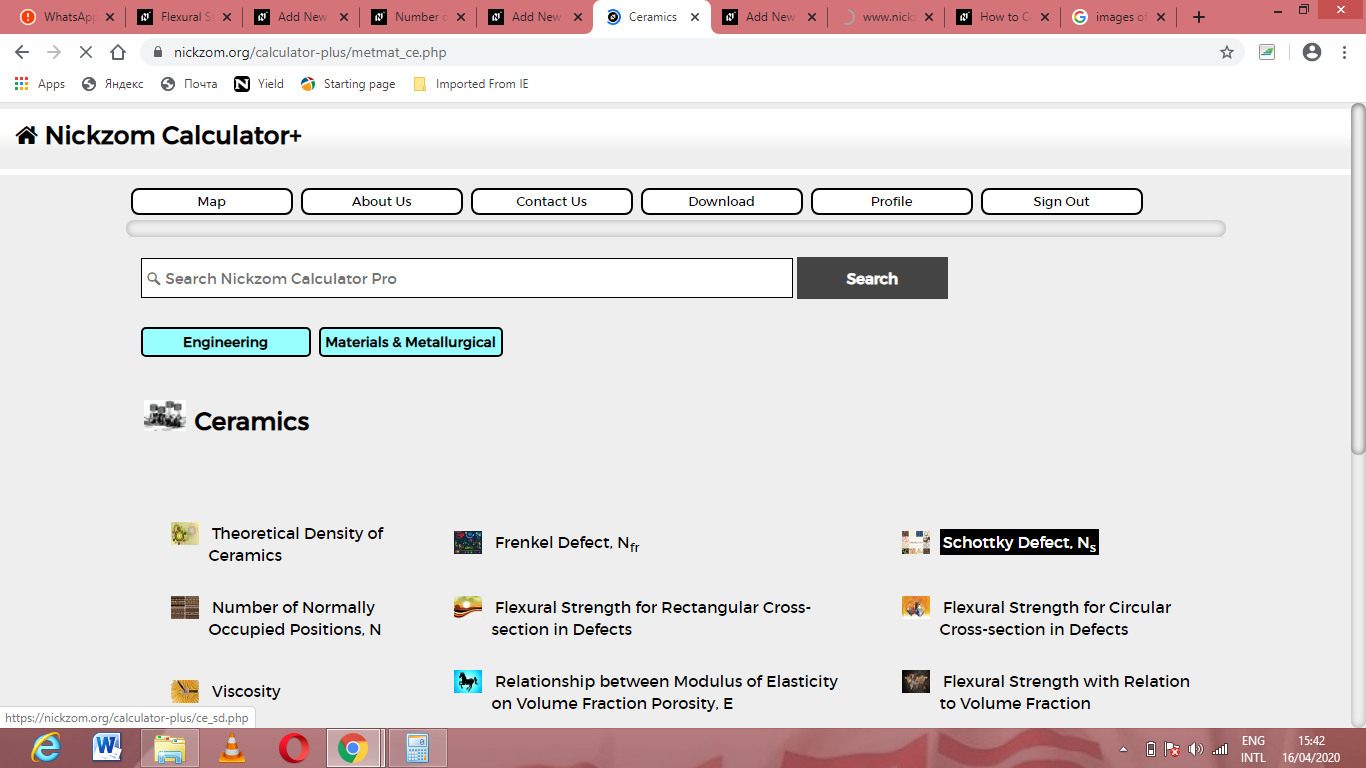The screenshot below displays the page or activity to enter your values, to get the answer for the schottky defect according to the respective parameters which are the N, activation energy (Qs), Boltzmann’s Constant (K) and temperature (T).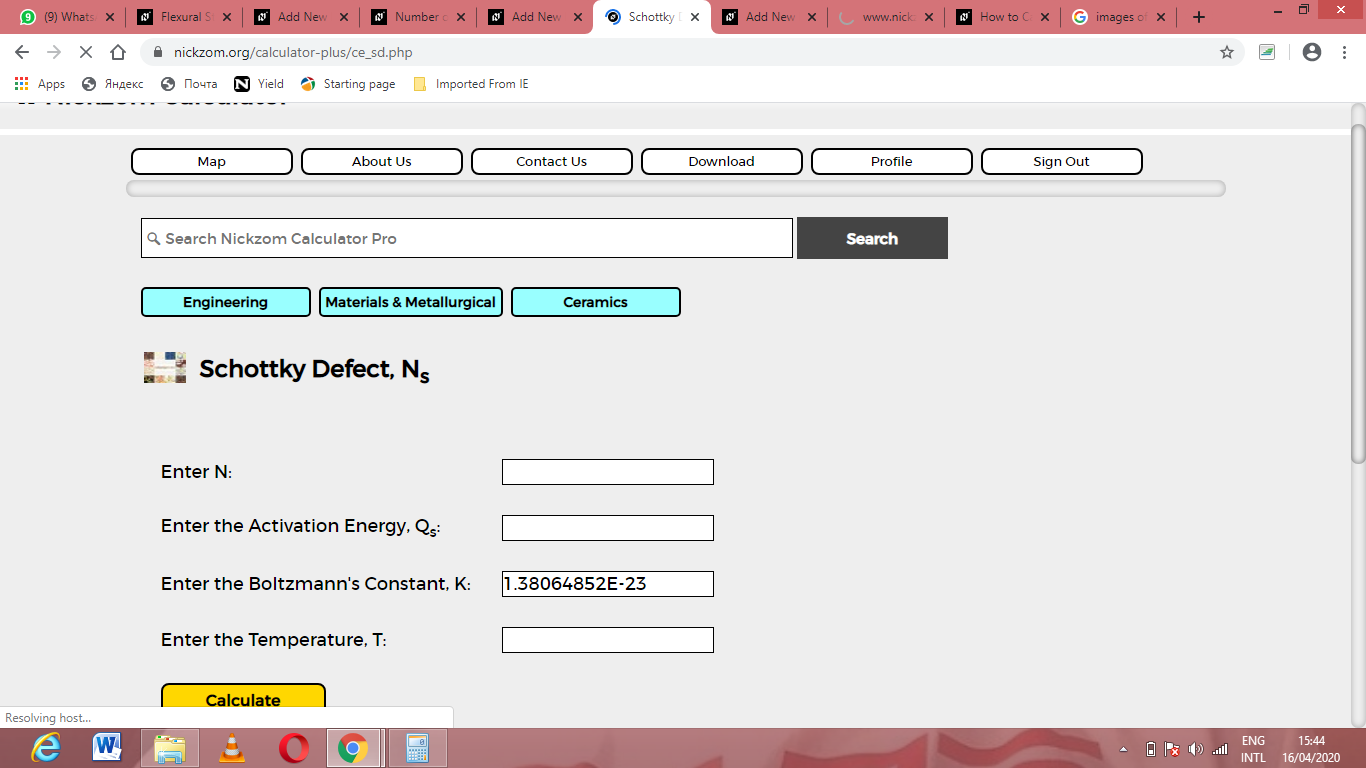Now, enter the values appropriately and accordingly for the parameters as required by the N is 22, activation energy (Qs) is 44, Boltzmann’s Constant (K) is 1.38064852e-23 and temperature (T) is 30.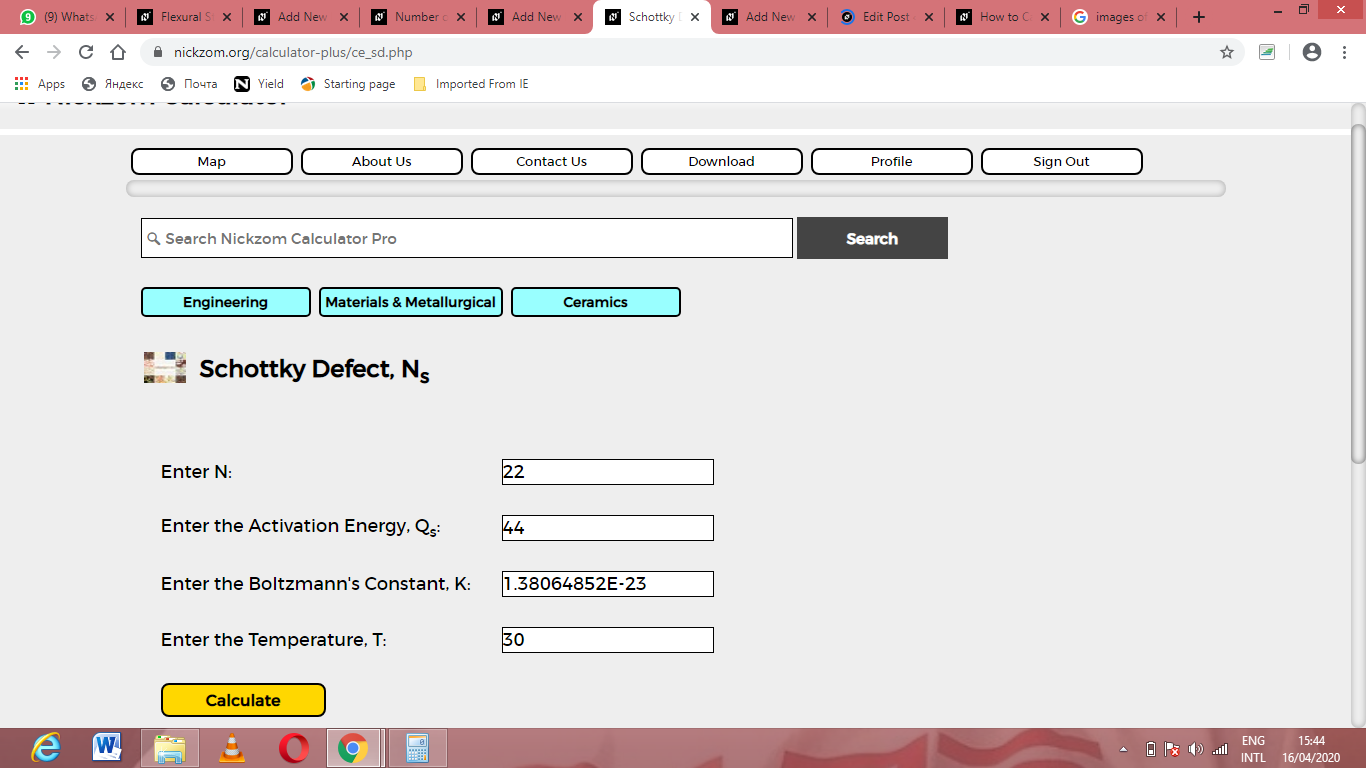Finally, Click on Calculate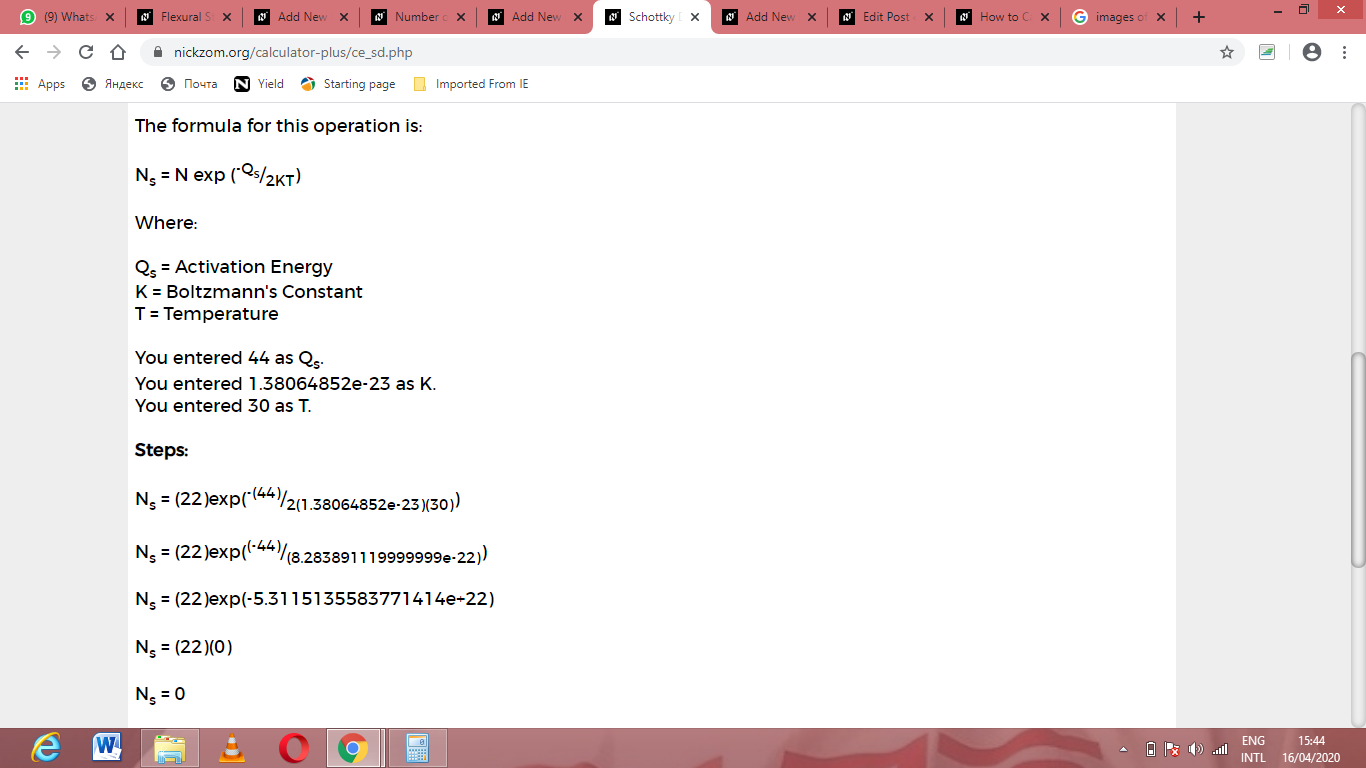As you can see from the screenshot above, Nickzom Calculator– The Calculator Encyclopedia solves for the schottky defect and presents the formula, workings and steps too.